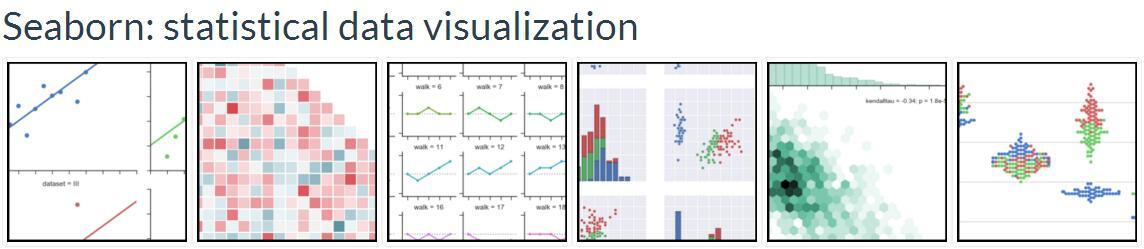## 绘制数据网格

``````%matplotlib inline
import numpy as np
import pandas as pd
import seaborn as sns
from scipy import stats
import matplotlib as mpl
import matplotlib.pyplot as plt

sns.set(style="ticks")
np.random.seed(sum(map(ord, "axis_grids")))``````

## 用FacetGrid子集数据

``````tips = sns.load_dataset("tips")

total_billtipsexsmokerdaytimesize016.991.01FemaleNoSunDinner 2110.341.66MaleNoSunDinner 3221.013.50MaleNoSunDinner 3323.683.31MaleNoSunDinner 2424.593.61FemaleNoSunDinner 4

``g = sns.FacetGrid(tips, col="time")``

``````g = sns.FacetGrid(tips, col="time")
g.map(plt.hist, "tip");``````

``````g = sns.FacetGrid(tips, col="sex", hue="smoker")
g.map(plt.scatter, "total_bill", "tip", alpha=.7)

``````g = sns.FacetGrid(tips, row="smoker", col="time", margin_titles=True)
g.map(sns.regplot, "size", "total_bill", color=".3", fit_reg=False, x_jitter=.1);``````

``````g = sns.FacetGrid(tips, col="day", size=4, aspect=.5)
g.map(sns.barplot, "sex", "total_bill");``````

``````titanic = sns.load_dataset("titanic")
titanic = titanic.assign(deck=titanic.deck.astype(object)).sort_values("deck")
g = sns.FacetGrid(titanic, col="class", sharex=False,
gridspec_kws={"width_ratios": [5, 3, 3]})
g.map(sns.boxplot, "deck", "age");``````

``````ordered_days = tips.day.value_counts().index
g = sns.FacetGrid(tips, row="day", row_order=ordered_days,
size=1.7, aspect=4,)
g.map(sns.distplot, "total_bill", hist=False, rug=True);``````

``````pal = dict(Lunch="seagreen", Dinner="gray")
g = sns.FacetGrid(tips, hue="time", palette=pal, size=5)
g.map(plt.scatter, "total_bill", "tip", s=50, alpha=.7, linewidth=.5, edgecolor="white")

``````g = sns.FacetGrid(tips, hue="sex", palette="Set1", size=5, hue_kws={"marker": ["^", "v"]})
g.map(plt.scatter, "total_bill", "tip", s=100, linewidth=.5, edgecolor="white")

``````attend = sns.load_dataset("attention").query("subject <= 12")
g = sns.FacetGrid(attend, col="subject", col_wrap=4, size=2, ylim=(0, 10))
g.map(sns.pointplot, "solutions", "score", color=".3", ci=None);``````

``````with sns.axes_style("white"):
g = sns.FacetGrid(tips, row="sex", col="smoker", margin_titles=True, size=2.5)
g.map(plt.scatter, "total_bill", "tip", color="#334488", edgecolor="white", lw=.5);
g.set_axis_labels("Total bill (US Dollars)", "Tip");
g.set(xticks=[10, 30, 50], yticks=[2, 6, 10]);

``````g = sns.FacetGrid(tips, col="smoker", margin_titles=True, size=4)
g.map(plt.scatter, "total_bill", "tip", color="#338844", edgecolor="white", s=50, lw=1)
for ax in g.axes.flat:
ax.plot((0, 50), (0, .2 * 50), c=".2", ls="--")
g.set(xlim=(0, 60), ylim=(0, 14));``````

## 将自定义函数应用在网格上

1. 必须绘制在“当前活动”的matplotlib轴上。 这对matplotlib.pyplot命名空间中的函数是正确的，如果要使用其方法，可以调用plt.gca来获取对当前Axes的直接引用。
2. 它必须接受它在位置参数中绘制的数据。 在内部，FacetGrid将传递一系列针对传递给FacetGrid.map()的命名位置参数的数据。
3. 它必须能够接受color和label关键字参数，理想情况下它将非常有用。在大多数情况下，使用一个通用的**kwargs字典是最简单的，并将其传递给底层的绘图函数。

``````def quantile_plot(x, **kwargs):
qntls, xr = stats.probplot(x, fit=False)
plt.scatter(xr, qntls, **kwargs)

g = sns.FacetGrid(tips, col="sex", size=4)
g.map(quantile_plot, "total_bill");``````

``````def qqplot(x, y, **kwargs):
_, xr = stats.probplot(x, fit=False)
_, yr = stats.probplot(y, fit=False)
plt.scatter(xr, yr, **kwargs)

g = sns.FacetGrid(tips, col="smoker", size=4)
g.map(qqplot, "total_bill", "tip");``````

``````g = sns.FacetGrid(tips, hue="time", col="sex", size=4)
g.map(qqplot, "total_bill", "tip")

``````g = sns.FacetGrid(tips, hue="time", col="sex", size=4,
hue_kws={"marker": ["s", "D"]})
g.map(qqplot, "total_bill", "tip", s=40, edgecolor="w")

``````def hexbin(x, y, color, **kwargs):
cmap = sns.light_palette(color, as_cmap=True)
plt.hexbin(x, y, gridsize=15, cmap=cmap, **kwargs)

with sns.axes_style("dark"):
g = sns.FacetGrid(tips, hue="time", col="time", size=4)
g.map(hexbin, "total_bill", "tip", extent=[0, 50, 0, 10]);``````

## 用PairGrid and pairplot()绘制成对的关系

PairGrid还允许您使用相同的绘图类型快速绘制小子图的网格，以在每个图形中显示数据。在一个PairGrid中，每个行和列分配给一个不同的变量，所以生成的图显示了数据集中的每个成对关系。这种风格的绘图有时被称为“散点图矩阵”，因为这是显示每个关系的最常见方式，但是PairGrid不仅限于散点图。

``````iris = sns.load_dataset("iris")
g = sns.PairGrid(iris)
g.map(plt.scatter);``````

``````g = sns.PairGrid(iris)
g.map_diag(plt.hist)
g.map_offdiag(plt.scatter);``````

``````g = sns.PairGrid(iris, hue="species")
g.map_diag(plt.hist)
g.map_offdiag(plt.scatter)

``````g = sns.PairGrid(iris, vars=["sepal_length", "sepal_width"], hue="species")
g.map(plt.scatter);``````

``````g = sns.PairGrid(iris)
g.map_upper(plt.scatter)
g.map_lower(sns.kdeplot, cmap="Blues_d")
g.map_diag(sns.kdeplot, lw=3, legend=False);``````

``````g = sns.PairGrid(tips, y_vars=["tip"], x_vars=["total_bill", "size"], size=4)
g.map(sns.regplot, color=".3")
g.set(ylim=(-1, 11), yticks=[0, 5, 10]);``````

``````g = sns.PairGrid(tips, hue="size", palette="GnBu_d")
g.map(plt.scatter, s=50, edgecolor="white")

PairGrid是灵活的，但是要快速查看一个数据集，可以使用pairplot()更容易。 默认情况下，该功能使用散点图和直方图，但是还可以添加其他几种（目前还可以绘制对角线上的对角线和KDEs的回归图）。

``sns.pairplot(iris, hue="species", size=2.5);``

``g = sns.pairplot(iris, hue="species", palette="Set2", diag_kind="kde", size=2.5)``

【全文完】

【未禾原创，所有章节已经完结，其他章节请点击跳转】

Seaborn(sns)官方文档学习笔记（第一章 艺术化的图表控制） - 知乎专栏

Seaborn(sns)官方文档学习笔记（第二章 斑驳陆离的调色板） - 知乎专栏

Seaborn(sns)官方文档学习笔记（第三章 分布数据集的可视化） - 知乎专栏

Seaborn(sns)官方文档学习笔记（第四章 线性关系的可视化） - 知乎专栏

Seaborn(sns)官方文档学习笔记（第五章 分类数据的绘制） - 知乎专栏

Seaborn(sns)官方文档学习笔记（第六章 绘制数据网格） - 知乎专栏